Welcome to part-5 of the series. We will start new question regarding new features which were released as a part of ES6.

Question 27- What are Maps and WeakMaps?Answer- Maps and WeakMaps are data structures that were introduced in ES6. They are similar to data structure Objects but one of the main features of Maps is that we can have Object as keys.

Let’s start with the problem with Objects. In the below example, we have an object “x” and another object “a”. Now we are using “a” as a key and push a value to “x”. Now, if we console log “x” the key is shown as [object object], but then also we can extract it’s value by x[a].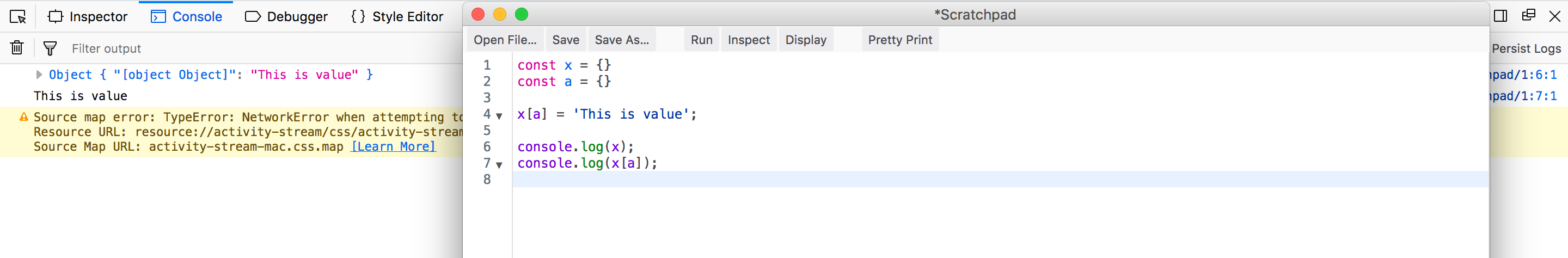Problem with Objects

But the real problem occurs when we add another object to “x” for key. It over-writes the earlier object. This occurs because in JavaScript we can have only 1 key as an object.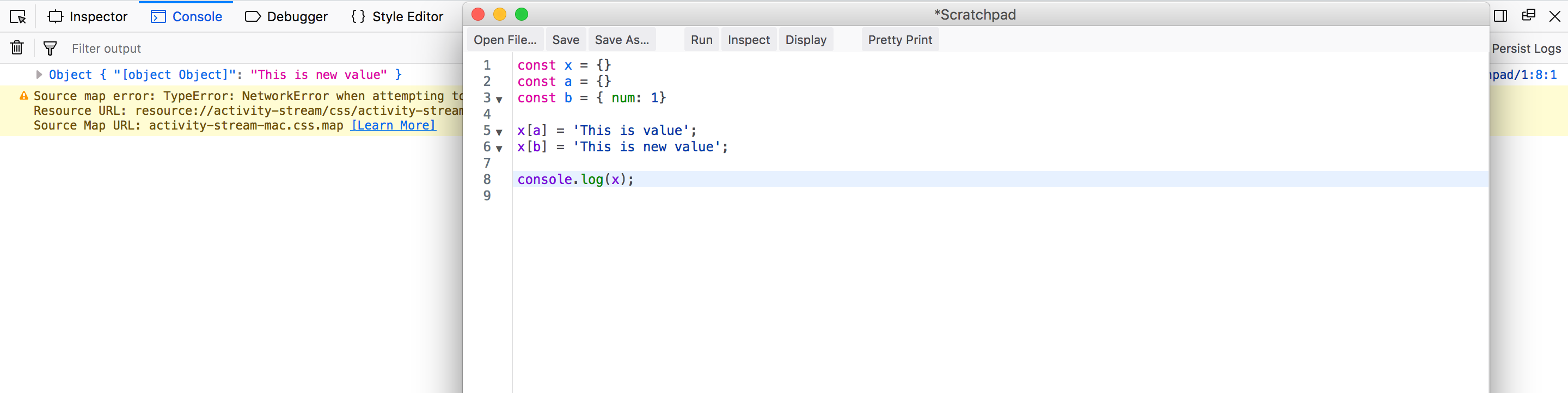Real Problem with Objects

Now we will look at how Maps help. We create Map by the new keyword. And also to add to Map we need use one of it’s method set. In the below example the objects “a” and “b” are been added as key successfully.

Notice that the proto object contains Symbol.iterator, so the for..of loop can be used to iterate over it like arrays. The same is not true for Objects.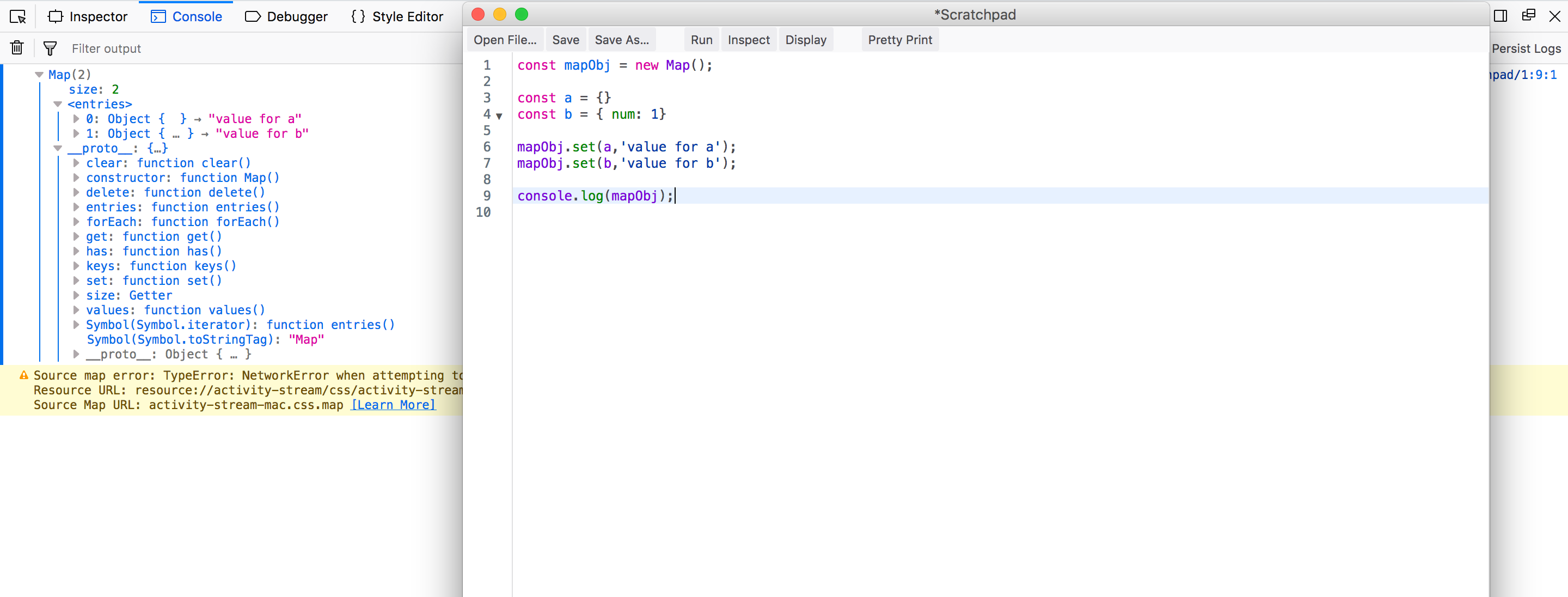Helpful Maps

We iterate over Map using the in-build method entries(). It returns an array of [key, value] for each element in Map. So, we de-structure it into [key, value] pair.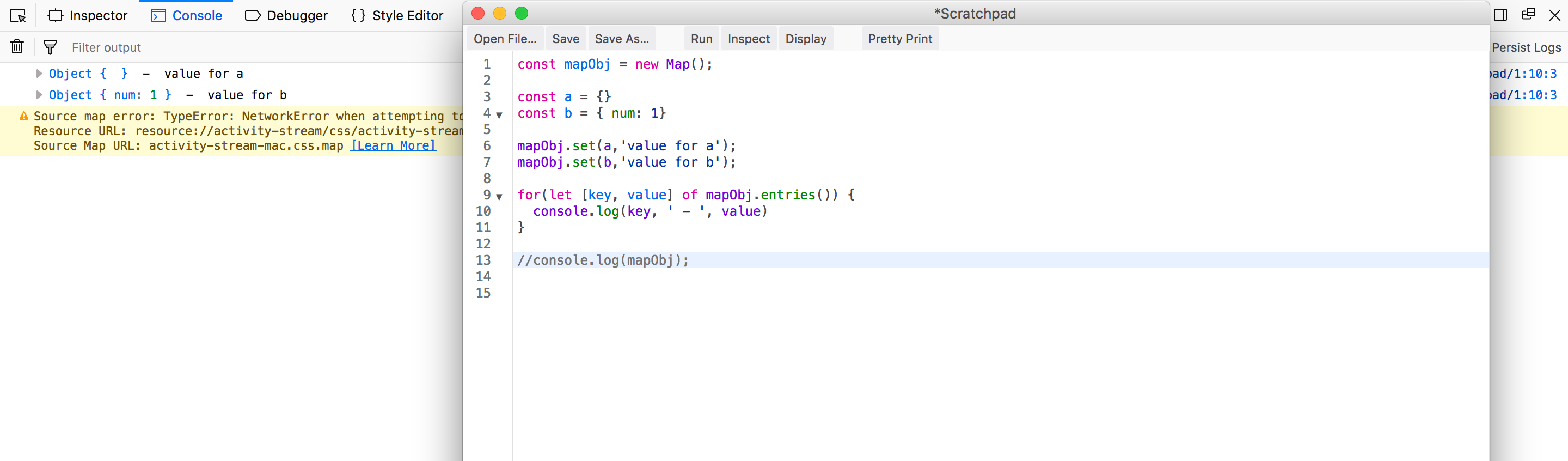iterate over Map

If we add the same object as key as in line 9 for “a”, the Map will over-write the old value of the key. Also, we can delete an entry by passing it’s key in delete method.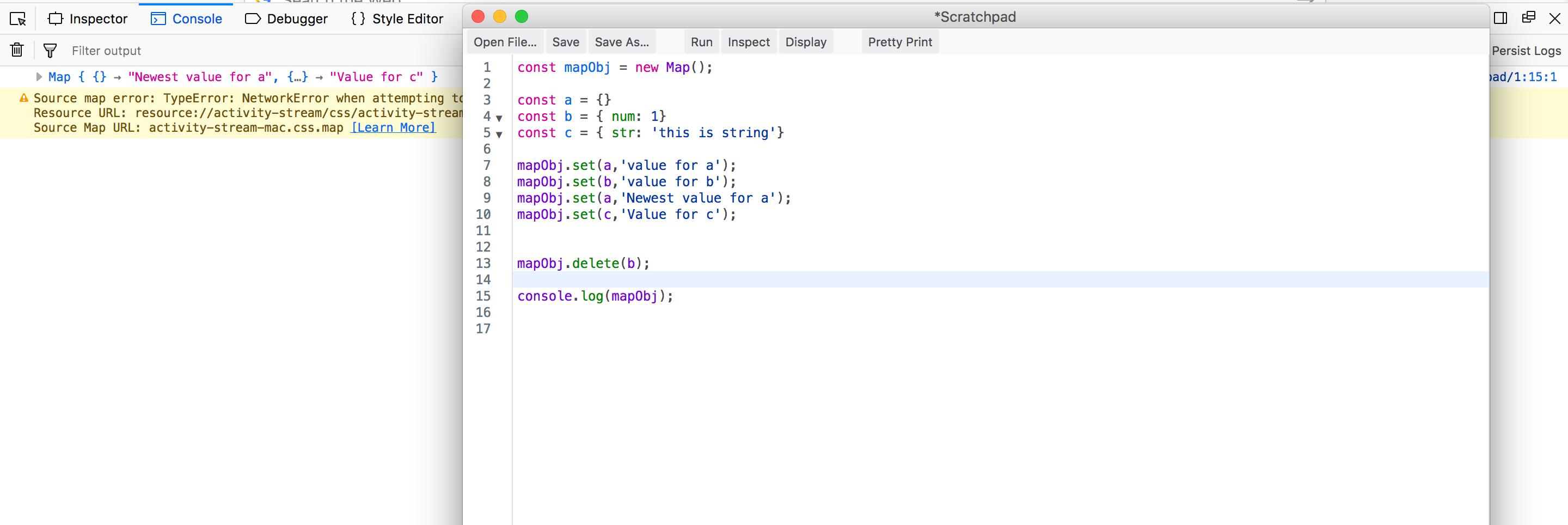delete and same key explained

There is one problem with Map and it is that it holds the Map’s key, even if the original key is deleted. That is the reason we use WeakMap. So, WeakMap allows garbage collector to work as it holds a weak reference to it’s key but Maps doesn’t allow garbage collector to work.

``````var k1 = {a: 1};
var k2 = {b: 2};

var map = new Map();
var wm = new WeakMap();

map.set(k1, 'k1 value');
wm.set(k2, 'k2 value');

k1 = null;
map.forEach(function (val, key) {
console.log(key,' - ', val); // Object { a: 1 }  -  k1 value
});

k2 = null;
console.log(wm.get(k2)); // undefined
``````

Another difference is that keys of WeakMaps are of type Object only. Primitive data types(Number, String, Boolean, Symbol) can only be used in Maps.

``````const m = new Map
m.set('a', 'b');

console.log(m); //Output - Map { a → "b" }

const w = new WeakMap;
w.set('a', 'b');

/*
Exception: TypeError: WeakMap key must be an object, got the string "a"
*/
``````

Question 28- What are Sets and WeakSets?Answer-Sets are also referenced data-types like arrays, but the key feature is that we cannot keep duplicate data. In a nutshell Sets are nothing but unique arrays.

Let consider below example. The way to create a Set is through the constructor function using “new” keyword. We add value to Set by using add method and delete value from Set using delete method.

Notice that it’s proto object have Symbol.iterator like array, which means it is iterable.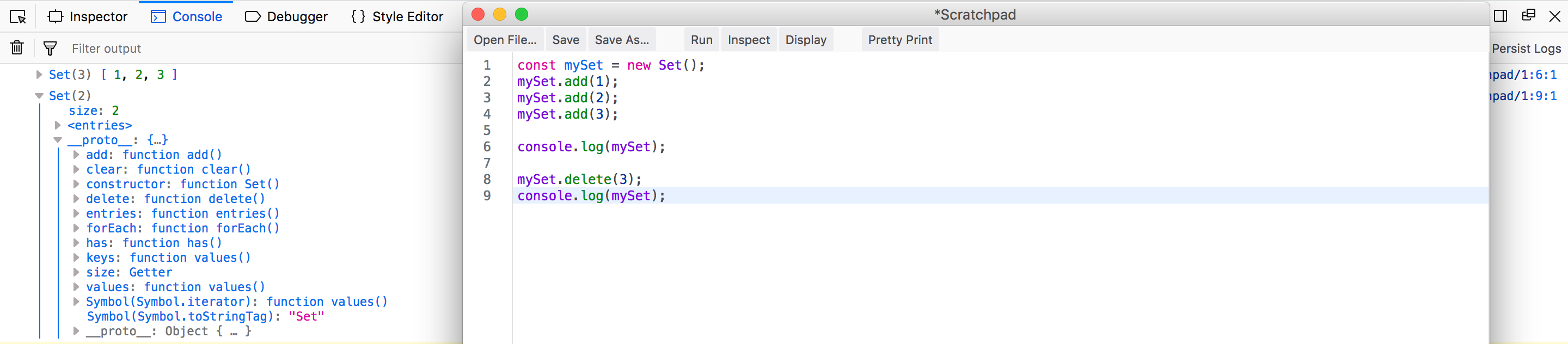Set creation

There is another method to create a Set. We can pass an iterable like array to the constructor function. Also, we cannot add duplicates value in a Set. Also we can add values to Set using add in method chaining format. All are shown in below example.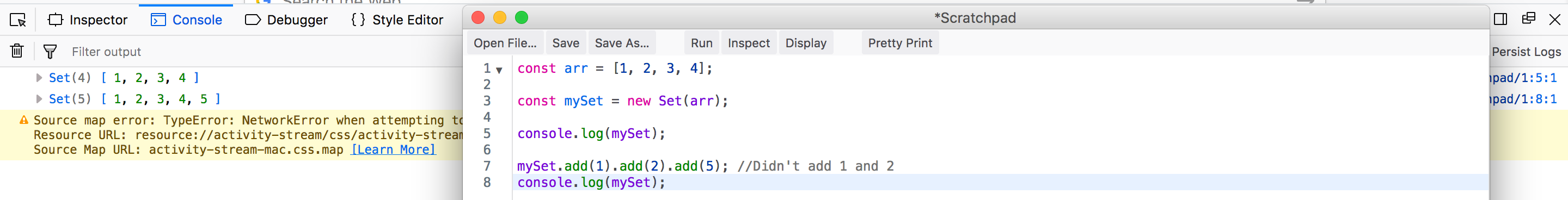No Dups are allowed

WeakSets are like WeakMaps and one of key feature is that we cannot have Primitive data-types(Number, String, Boolean, Symbol) as it’s items. So, the below will throw and error.

``````const ws = new WeakSet();

/*
Exception: TypeError: WeakSet value must be an object, got the number 1
*/
``````

So, we will add Objects to WeakSet. Also, notice that it’s proto doesn’t have many methods and it is not an iterable.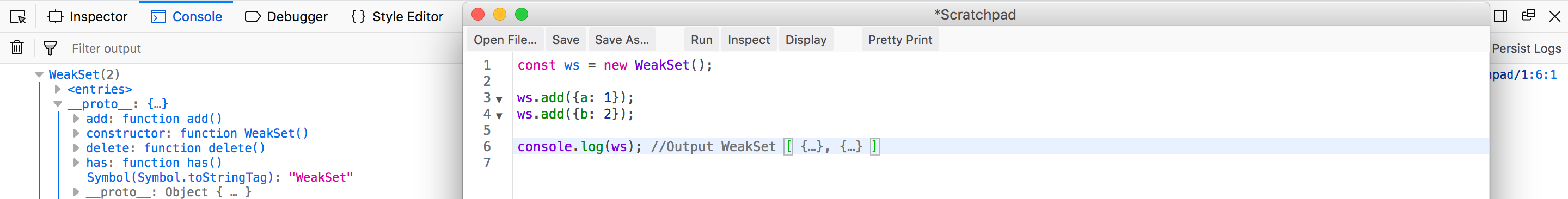WeakSet add elements

Question 29- What are Symbols primitive type in JavaScript?Answer-Symbols are the new primitive data-type introduced in ES6. Symbols is unique an immutable data-type. They are tokens that can be used as unique ids. Two Symbols are never the same, even if they are declared as same. Consider the below example.

``````let symbol1 = Symbol();
let symbol2 = Symbol();
console.log(symbol1 === symbol2); //Output - false
``````

We can also put some value inside the constructor in Symbol, which helps in identifying it. Also, the typeof is symbol

``````let symbol1 = Symbol('Symbol id: 1');

console.log(typeof symbol1); //Output - symbol
``````

We cannot directly concatenate it to a Sting and it will throw error.

``````let symbol1 = Symbol('Symbol id: 1');

console.log('We are concatenating '+ symbol1)
/*
Exception: TypeError: can't convert symbol to string
*/
``````

So, we have to convert a Symbol into a String like below.

``````let symbol1 = Symbol('Symbol id: 1');

console.log('We are concatenating - '+ symbol1.toString());
//Output - We are concatenating - Symbol(Symbol id: 1)
``````

One of the use case of Symbol is to be used as unique keys. We can use them very efficiently in Objects and Maps, where only unique keys are allowed.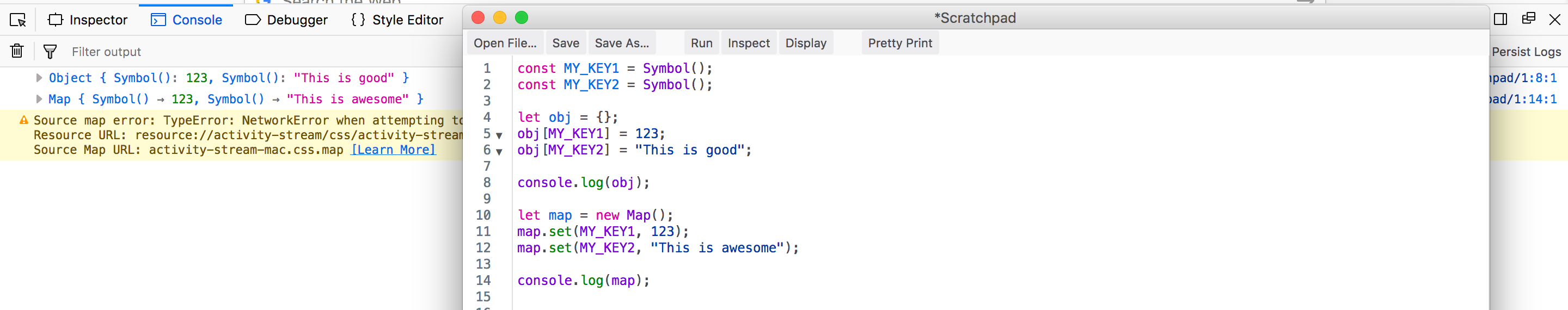unique keys

Question 30- What are template Strings in ES6?Answer-Template strings are the new way to write Strings in JavaScript and been introduced in ES6. We can now write Stings using back-ticks( ), which have a special way to interpolate variables.

Consider we want to show the famous Bond Line in our console. We declare a variable name and want to display it inside it.

The old JavaScript way is quite cumbersome and we have to use many + operators to concatenate Strings, whereas with back-ticks we interpolate variable name using \${}.

``````let name = "Bond";

let bondLine = "My name is " + name + ", James " + name;

console.log(bondLine); // My name is Bond, James Bond

let bondLineES6 = `
``````

My name is \${name}, James \${name} `;

``````console.log(bondLineES6); // My name is Bond, James Bond
``````

Question 31- What are default parameters in ES6?Answer-Default parameters are the parameters, which are used if we don’t pass any value to argument in a function.

We will see how we use to handle this situation before ES6, if user didn’t supplied a value to argument. So, we check inside the function “add” if “a” has a value or assign 0 to it. Same is done for “b”

``````var add = function(a, b){
a = a || 0;
b = b || 0;
return a + b;
}

//Passing no argument

//Passing argument 'a'

//Passing argument 'b'
``````

The same can be done in ES6 by changing the parameter to contain the default value. Re-writing the above with ES6 default parameters.

``````let add = (a=0, b=0) => {
return a + b;
}

//Passing no argument

//Passing argument 'a'

//Passing argument 'b'
``````

Question 32- What are Spread operator and Rest parameters?Answer-Just like plus(+) and minus(-) are operators, spread(…) is also an operator in JavaScript. Basically Spread operator spreads on demand.

When you pass arguments using Spread operators they are called Rest parameters.

Consider the below example for arrFunc. We can pass any number of arguments to a function and use the …arr to get it. Now inside the function the arr gets converted into and array. This functionality is quite similar to arguments object. Now see the restFunc, here we are using rest parameter to get the remaining of the arguments. We can get argument a, b, c and then the rest is convertd into array.

``````let arrFunc = (...arr) => {
console.log(arr);
}

arrFunc(1, 2, 3, 4, 5);
//Output - [ 1, 2, 3, 4, 5 ]

let restFunc = (a, b, c, ...n) => {
console.log(`
``````

a, b, c are- \${a} \${b} \${c} `);
console.log(n); }

``````restFunc(1, 2, 3, 4, 5, 9 , 8, 7);
//Output - a, b, c are- 1 2 3
//[ 4, 5, 9, 8, 7 ]
``````

The Spread operator can be use separately from function parameter and have some very useful use in array manipulation. They can now also be used with Objects.

Consider that we want to copy an array to other array. Now as you can see in the problem that when we assign variable y to x, we are referencing it and any changes to y will reflect in x. In the first solution, we use the new Javascript method Object.assign() to copy all contents of “a” to an empty array and then assigning it to “b”. But the better and simple solution is with Spread operator where we spread the array “c”, and take it’s content in an array.

``````//Problem
let x = [1, 2, 3, 4];
let y = x;
y.push(5);

console.log(y); // [ 1, 2, 3, 4, 5 ]
console.log(x); // [ 1, 2, 3, 4, 5 ]

//Solution
let a = [1, 2, 3, 4];
let b = Object.assign([], a);
b.push(9);

console.log(a); // [ 1, 2, 3, 4 ]
console.log(b); // [ 1, 2, 3, 4, 9 ]

let c = [1, 2, 3, 4, 10];
let d = [...c];
d.push(13);

console.log(c); // [ 1, 2, 3, 4, 10 ]
console.log(d); // [ 1, 2, 3, 4, 10, 13 ]
``````

One other use of Spread operator is to merge two or more arrays like below.

``````let x = [1, 2];
let a = [3, 4];
let c = [9, 10];
let d = [...x, ...a, ...c];

console.log(d); // [ 1, 2, 3, 4, 9, 10 ]
``````

Another use it that if we pass an array to argument of function or build in functions, which expects arguments. Consider the below examples.

``````let a = [1, 2, 3, 4];

//Passing array as arguments
let arrFunc = (...arr) => {
console.log(arr);
}

arrFunc(a); //[1, 2, 3, 4]

//Using in inbuild functions
console.log(Math.min(...a)); // 1
console.log(Math.max(...a)); // 4
console.log(Math.hypot(...a)); // 5.477225575051661
``````

We can also use Spread operator in Objects. This is practically used in reducer in React. So, by it we can do both clone and merge as shown in below example. Notice, that in mergedObj foo is “baz” as Object cannot have duplicate keys.

``````var obj1 = { foo: 'bar', x: 42 };
var obj2 = { foo: 'baz', y: 13 };

var clonedObj = { ...obj1 };
console.log(clonedObj); // Object { foo: "bar", x: 42 }

var mergedObj = { ...obj1, ...obj2 };
console.log(mergedObj); // Object { foo: "baz", x: 42, y: 13 }
``````

Question 33- Explain Destructuring in ES6?Answer-With the destructuring syntax, you can extract small fragments from arrays an objects. Destructuring syntax can be used in variable declaration or variable assignment. You can also handle nested structure by using nested destructuring syntax.

Object Destructuring Consider the below example. Basically, you use an object literal on the left-hand-side of an assignment expression for object destructuring.

``````const developer = {
firstname: 'Nabendu',
lastname: 'Biswas',
country: 'India'
};

// Object Destructuring
const { firstname, lastname, country } = developer;

console.log(firstname, lastname, country); // Nabendu Biswas India
``````

Default Values You can pass default values to be assigned to variables in which no values are passed.

``````const person = {
name: 'Nabendu Biswas',
country: 'India'
};

// Assign default value of 25 to age if undefined
const { name, country, age = 25 } = person;

// Here I am using ES6 template literals
console.log(`
``````

I am \${name} from \${country} and I am \${age} years old. `);

``````// I am Nabendu Biswas from India and I am 25 years old.
``````

Nested Object Destructuring If there is nested object as in below case, we can destructure it by adding it’s value to another object syntax

``````const student = {
name: 'Hriday Biswas',
age: 5,
scores: {
maths: 74,
english: 63
}
};

const { name, age, scores: {maths, english} } = student;

console.log(`
``````

\${name} who is \${age} years old, scored \${maths} in Maths and \${english} in English. `);

``````// Hriday Biswas who is 5 years old, scored 74 in Maths and 63 in English.
``````

Array Destructuring It is similar to object destructuring, but here instead of keys you assign any variable.

``````const rgb = [255, 200, 0];

// Array Destructuring
const [red, green, blue] = rgb;

console.log(`
``````

R: \${red}, G: \${green}, B: \${blue} `); // R: 255, G: 200, B: 0

Skipping Items It is possible to skip items by omitting items with comma(,).

``````//Example 1
const rgb = [200, 255, 100];

// Skip the first two items
// Assign the only third item to the blue variable
const [,, blue] = rgb;

console.log(`
``````

Blue: \${blue} `); // Blue: 100

``````//Example 2
const hex = ['# fff', '# 000', '# 678'];

// Skip the first and last items
// Assign the only second item to the black variable
const [, black,] = hex;

console.log(`
``````

Black: \${black}`); // Black: # 000

Spread Items We can use Spread Operator(…) to capture rest of the items, after destructuring some. Consider the below example.

``````const rainbow = ['red', 'orange', 'yellow', 'green', 'blue', 'indigo', 'violet'];

// Assign the third items to yellow
// Assign the remaining items to otherColors variable using the spread operator(...)
const [,, yellow, ...otherColors] = rainbow;

console.log(otherColors); // [ "green", "blue", "indigo", "violet" ]
``````

Destructured Function Parameters Destructuring can also be applied on function parameters to extract values and assign them to local variables.

``````const student1 = {
name: 'Hriday Biswas',
age: 5,
scores: {
maths: 74,
english: 63,
science: 85
}
};

const student2 = {
name: 'Ganavi',
age: 6,
scores: {
english: 34,
science: 54
}
};

// Destructuring with default value for scores.maths
function displaySummary({name, age, scores: { maths = 10, english, science }}) {
console.log('Hello, ' + name);
console.log('Aged, ' + age);
console.log('Your Maths score is ' + maths);
console.log('Your English score is ' + english);
console.log('Your Science score is ' + science);
}

displaySummary(student1);
displaySummary(student2);
``````

This concludes part-5 of the series.

This post is also available on DEV.